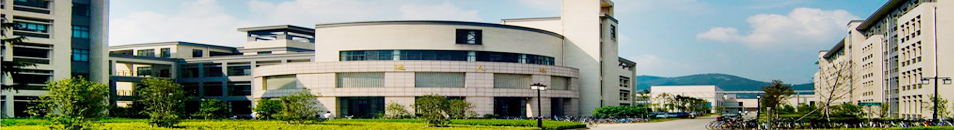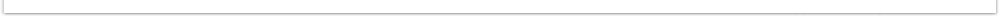﻿ 3月22日讲座——俄罗斯科学院Sobolev数学研究所系列学术讲座之一-江南大学 理学院### 3月22日讲座——俄罗斯科学院Sobolev数学研究所系列学术讲座之一

IAbelian symmetries in multi-Higgs-doublet models

Since birth the group theory established deep connections with distinct areas of mathematics and other sciences. In 2008 the famous Abelian prize in mathematics was given to two specialists in groups theory for their progress in solving the classification of finite simple groups problem.In the talk we discuss recent progress in physics of elementary particles obtained by using the power of group theory.

IIPeriodic groups whose element orders are small

Consider a periodic group G, and the set of its element orders ω(G), which is called spectrum. Assume that ω(G) is given. We discuss conditions, which guarantee that a corresponding group G is locally finite, i.e. each its finite subset generates a finite subgroup. In particular, we discuss the problem of recognizing nonabelian finite simple groups by spectrum in the class of periodic groups.